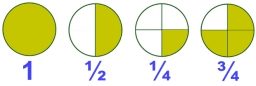# Eq-frac

Solve the following equation with fractions:

h + 1/3 =5/3

h =  1.3333

### Step-by-step explanation:

h + 1/3 =5/3

3h = 4

h = 4/3 ≈ 1.333333

Our simple equation calculator calculates it.Did you find an error or inaccuracy? Feel free to write us. Thank you!Tips to related online calculators
Need help to calculate sum, simplify or multiply fractions? Try our fraction calculator.
Do you have a linear equation or system of equations and looking for its solution? Or do you have a quadratic equation?

## Related math problems and questions:

• Fraction eq2/3x + 5/7 = 1/2x + 22/21
• Fractions and mixed numerals(a) Convert the following mixed numbers to improper fractions. i. 3 5/8 ii. 7 7/6 (b) Convert the following improper fraction to mixed number. i. 13/4 ii. 78/5 (c) Simplify these fractions to their lowest terms. i. 36/42 ii. 27/45 2. evaluate following ex
• Fraction + eqSolve following simple equation with fractions: -5/6(8+5b) = 75 + 5/3b
• Equation with fractionsSolve an equation: 1- (2x-5)/(6) =3- (x)/(4) It is an equation with fractions.
• Equation with xSolve the following equation: 2x- (8x + 1) - (x + 2) / 5 = 9
• One thirdIf 3/5 is 360, how much is 1/3?
• EqnSolve equation with fractions: 2x/3-50=40+x/4
• EquationSolve equation and check the result: 1.4x - 3/2 + x - 9,8 = x + 0,4/3 - 7 + 1,6/6
• Reciprocal equation 3Solve reciprocal equation: 1/2 + 2/3=1/x
• Simple equationSolve the following simple equation: 2. (4x + 3) = 2-5. (1-x)
• EquationFind x: x + 1/2 = 1/3
• Simple equation 5Solve equation with fractions: X × 3/8 = 1/2
• What is 9What is the value of x in the proportion 2 and one-fourth over x = 1 and one-half over 3 and three-fifths? 2 and two-fifths 5 and two-fifths 8 and 1 over 10 12 and 3 over 20
• Simplify 7Simplify. 7-1/3÷ 3-2/3 of 2+ 4-1/2÷ 2-1/4+ 1/2 solution and by step by step
• Equation 25Solve following simple equation: 3/4(x+5)=1/2(x+9)
• Fraction of a NumberIf 1/2 of 1/3 of 1/4 of 1/5 of a number is 2.5. what is the number?
• Evaluate expressionEvaluate expression using BODMAS rule: 1 1/4+1 1/5÷3/5-5/8